# 陶博士2006，所有秘公式汇总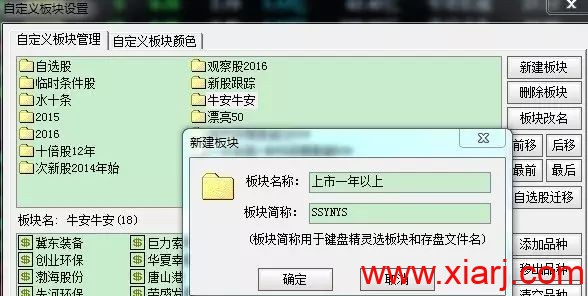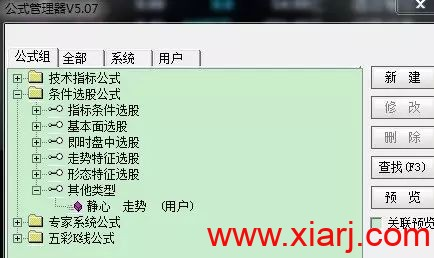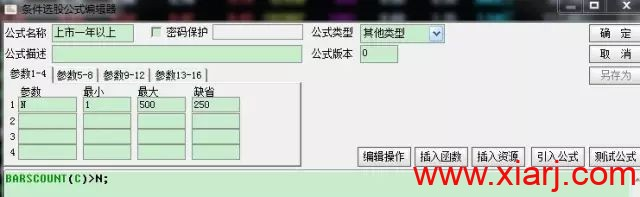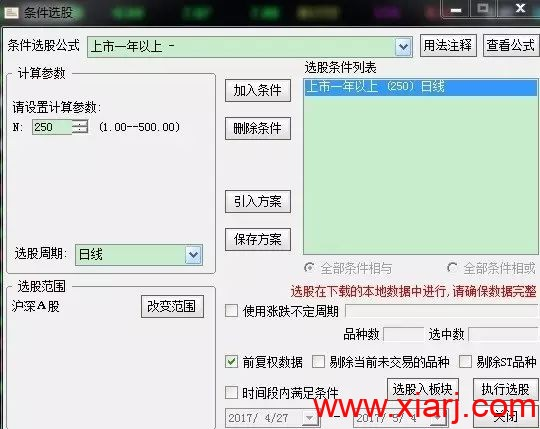EXTRS:(C-REF(C,N))/REF(C,N);

N缺省值为250。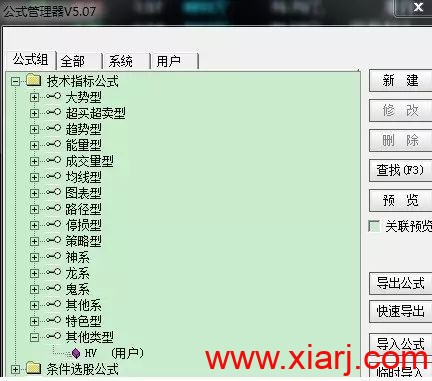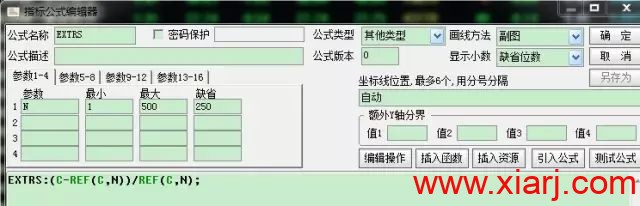1.选中第1条扩展数据，如下图：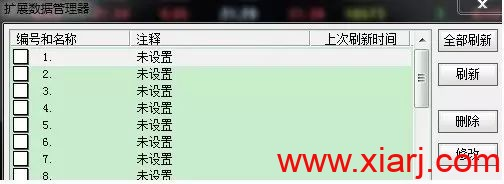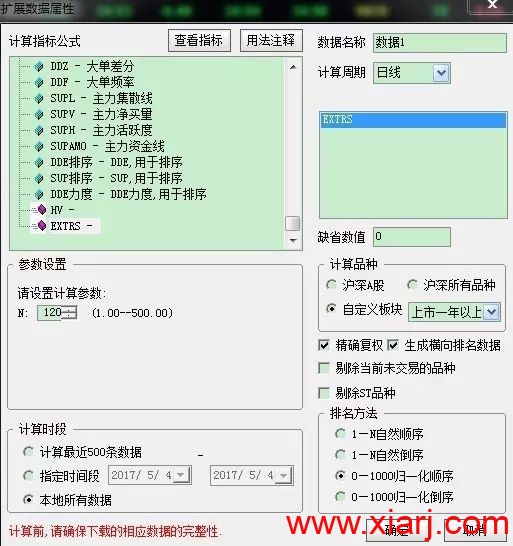2.再重复上述步骤，  在扩展数据属性里首先设置数据2，计算参数为250（对应一年期的RPS）。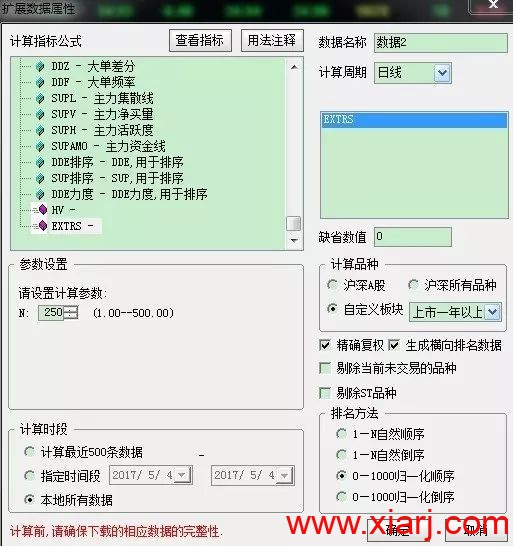3.再重复上述步骤，  在扩展数据属性里首先设置数据3，计算参数为50（对应50日的RPS）。

4. 在在在再重复上述步骤，  在扩展数据属性里首先设置数据“10”，计算参数为20（对应20日的RPS）。（因为很多朋友都设置过行业RPS，注意看好你的扩展数据“数值是多少”到底是不是10，不是的话要不自己改一下，要不从新跟我做一遍）因为我设置过细分行业跟板块行业所以我的是数据10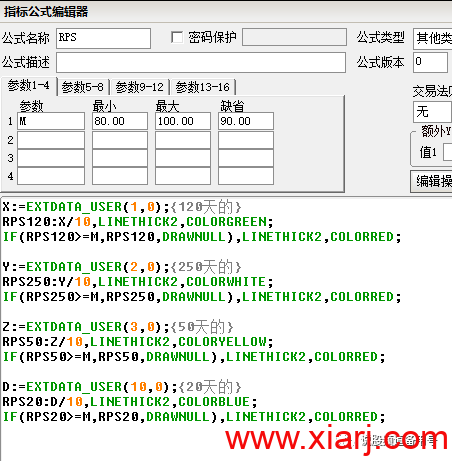X:=EXTDATA_USER(1,0);{120天的}

RPS120:X/10,LINETHICK2,COLORGREEN;

IF(RPS120>=M,RPS120,DRAWNULL),LINETHICK2,COLORRED;

Y:=EXTDATA_USER(2,0);{250天的}

RPS250:Y/10,LINETHICK2,COLORWHITE;

IF(RPS250>=M,RPS250,DRAWNULL),LINETHICK2,COLORRED;

Z:=EXTDATA_USER(3,0);{50天的}

RPS50:Z/10,LINETHICK2,COLORYELLOW;

IF(RPS50>=M,RPS50,DRAWNULL),LINETHICK2,COLORRED;

D:=EXTDATA_USER(10,0);{20天的}

RPS20:D/10,LINETHICK2,COLORBLUE;

IF(RPS20>=M,RPS20,DRAWNULL),LINETHICK2,COLORRED;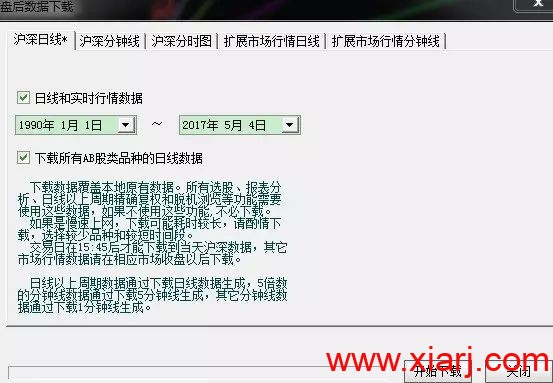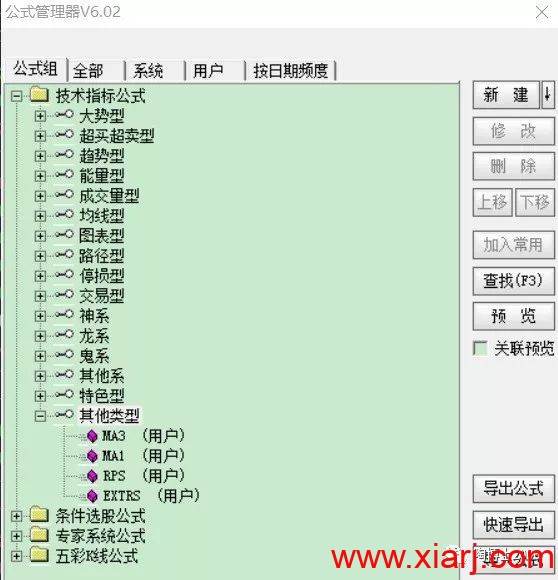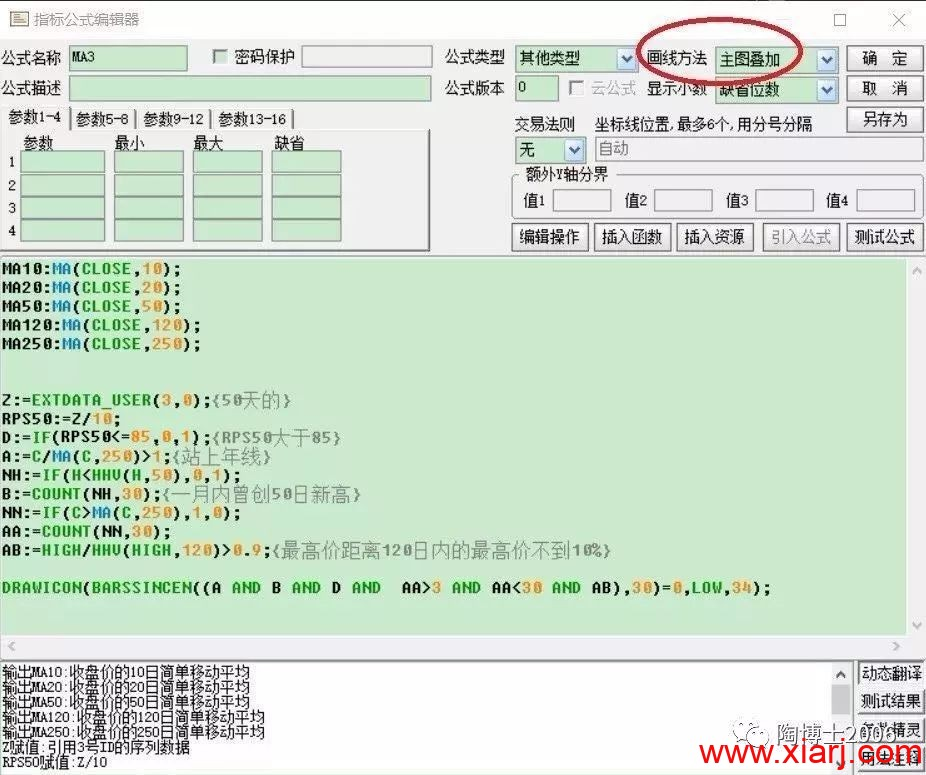MA10:MA(CLOSE,10);

MA20:MA(CLOSE,20);

MA50:MA(CLOSE,50),COLORRED,LINETHICK3;

MA120:MA(CLOSE,120);

MA250:MA(CLOSE,250),COLORYELLOW,LINETHICK2;

Z:=EXTDATA_USER(3,0);{50天的}

RPS50:=Z/10;

D:=IF(RPS50<=85,0,1);{RPS50大于85}

A:=C/MA(C,250)>1;{站上年线}

NH:=IF(H<HHV(H,50),0,1);

B:=COUNT(NH,30);{一月内曾创50日新高}

NN:=IF(C>MA(C,250),1,0);

AA:=COUNT(NN,30);

AB:=HIGH/HHV(HIGH,120)>0.9;{最高价距离120日内的最高价不到10%}

DRAWICON(BARSSINCEN((A AND B AND D AND  AA>2  AND AA<30 AND AB),30)=0,LOW,34);

ZZ:=EXTDATA_USER(10,0);

RPS20:=ZZ/10;

DD:=IF(RPS20<=97,0,1);

A1:=C/MA(C,250)>1;

NH1:=IF(H<HHV(H,20),0,1);

B1:=COUNT(NH1,30);

NN1:=IF(C>MA(C,250),1,0);

AA1:=COUNT(NN1,30);

AB1:=HIGH/HHV(HIGH,120)>0.9;

DRAWICON(BARSSINCEN(( A1 AND AB1 AND AA1>2  AND    DD AND   C<MA(C,10)),30)=0,LOW,9);

X:=CONST(HHV(HIGH,150));

X2:=HHVBARS(H,150)+1;

X3:=CONST(LLV(LOW,X2));

X4:=X3/X;X5:=X4*100-100;DRAWNUMBER(L=X3,X3,X5);

C2:=REF(C,1);

STICKLINE(涨停,OPEN,CLOSE,2,0),COLORYELLOW;

{黄色涨停}

STICKLINE(涨停,H,L,0,0),COLORRED;

STICKLINE(跌停,OPEN,CLOSE,2,0),COLORBLUE;{跌停}

STICKLINE(跌停,H,L,0,1),COLORBLUE;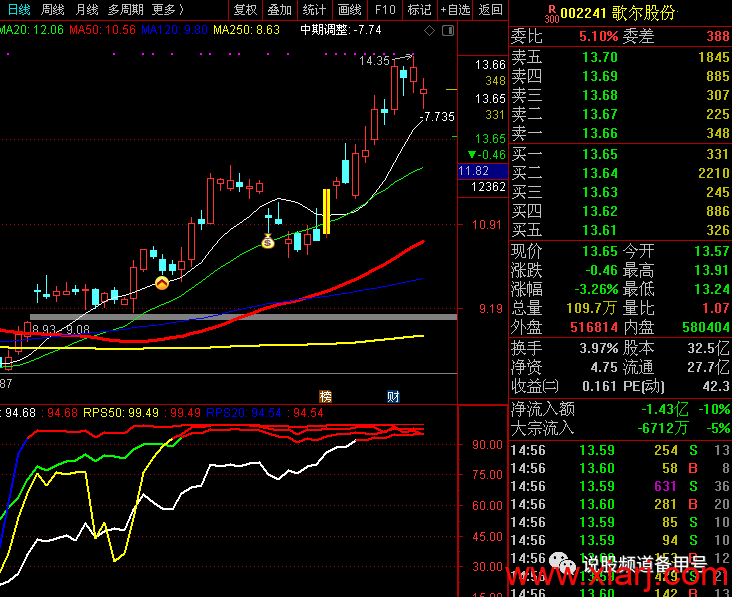Z:=EXTDATA_USER(3,0);{50天的}

RPS50:=Z/10;

D:=IF(RPS50>=85,1,0);{RPS50大于85}

A:=C/MA(C,250)>1;{站上年线}

NH:=IF(H<HHV(H,50),0,1);

B:=COUNT(NH,30);{一月内曾创50日新高}

NN:=IF(C>MA(C,250),1,0);

AA:=COUNT(NN,30);

AB:=HIGH/HHV(HIGH,120)>0.9;{最高价距离120日内的最高价不到10%}

A AND B AND D AND AA>2 AND AA<30 AND AB;{选出刚刚站上年线小于30天，大于2天的};

通达信软件，输入：.905（注意905前面有一个点），或者按Control+T，进入条件选股界面：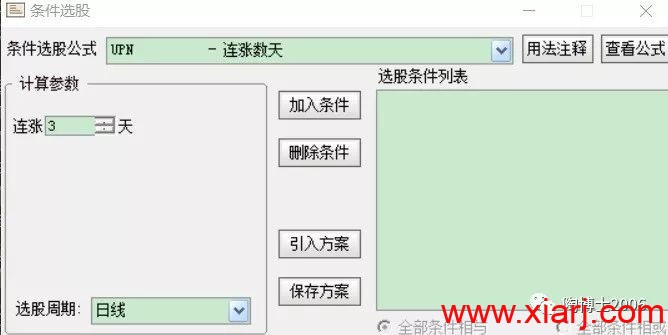点击：加入条件，再点击：保存方案，再点击：确定（这样做的目的以后只需要点击引入方案，就可以添加这个“月线反转”的选股条件）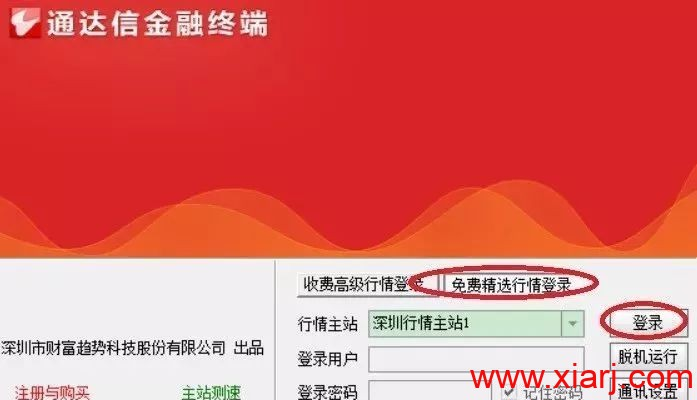Control+F，进入如下界面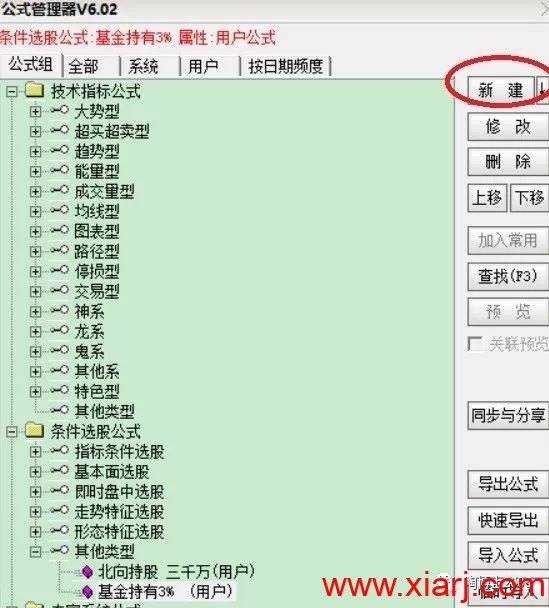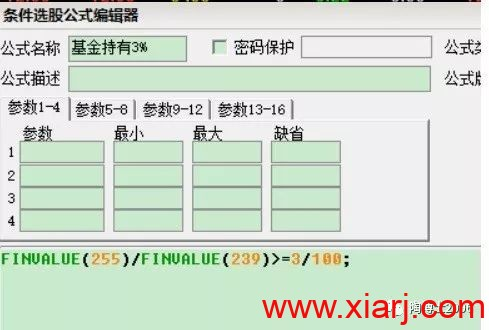FINVALUE(255)/FINVALUE(239)>=3/100;

GPJYVALUE(6,1,1)*C>30000000;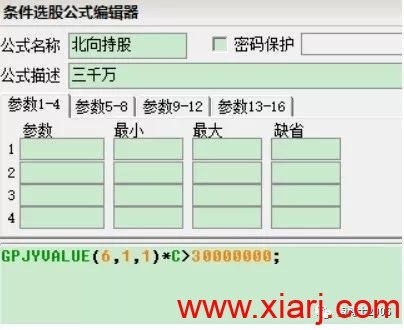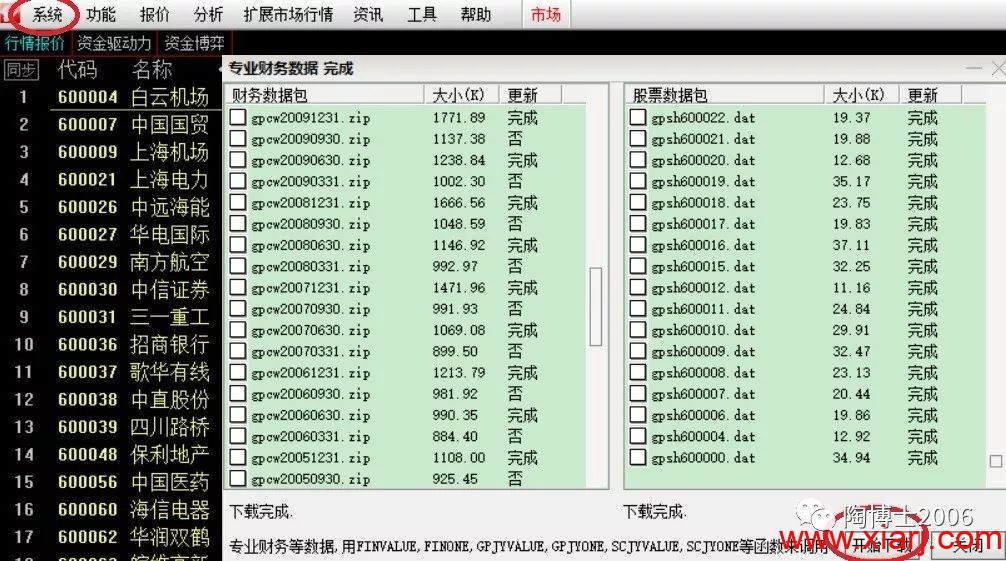Control+T，开始选股：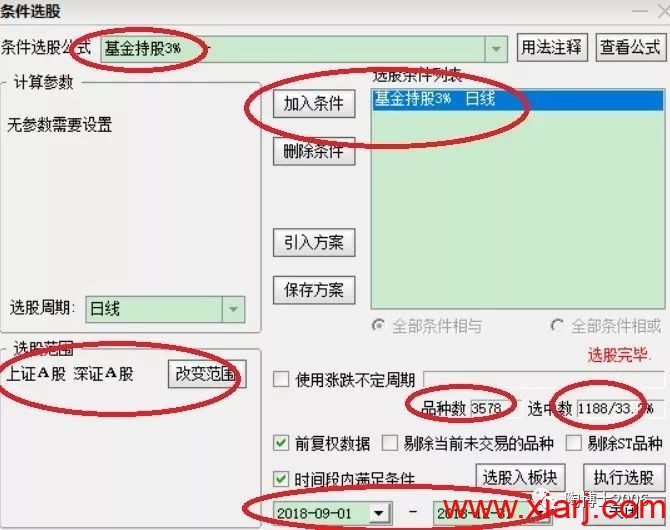Control+T，开始选股：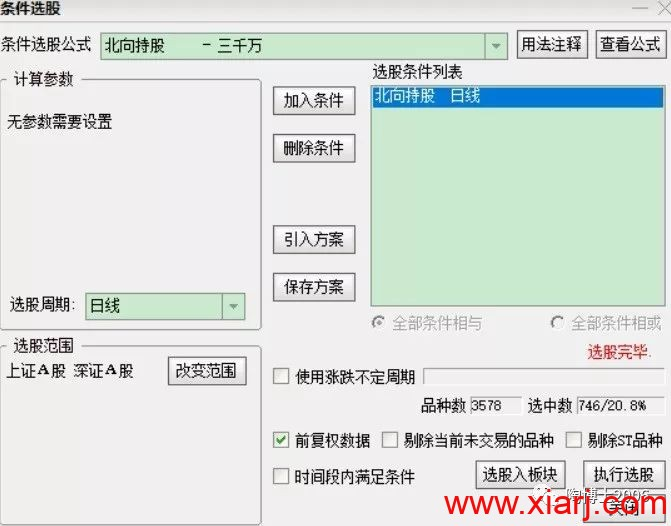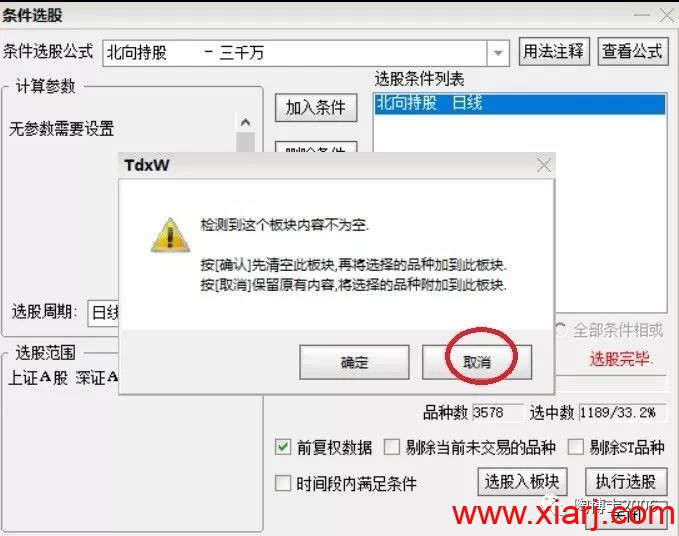“基金持股3%”（1189只）与“北向持股三千万”（746只），合并起来“基金持股3%+北向持股三千万”（1433只），因为有部分股票是重叠的。

这个“基金持股3%+北向持股三千万”（1433只），目前是我所有选股工作的第一步。

ZZ:=EXTDATA_USER(10,0);

RPS20:=ZZ/10;

DD:=IF(RPS20<=97,0,1);

A1:=C/MA(C,250)>1;

NH1:=IF(H<HHV(H,20),0,1);

B1:=COUNT(NH1,30);

NN1:=IF(C>MA(C,250),1,0);

AA1:=COUNT(NN1,30);

AB1:=HIGH/HHV(HIGH,120)>0.9;

DRAWICON(BARSSINCEN(( A1 AND AA1>2  AND    AB1 AND  DD AND   C<MA(C,10)),30)=0,LOW,9);点击：加入条件，再点击：保存方案，再点击：确定（这样做的目的以后只需要点击引入方案，就可以添加这个“捡钱”的选股条件）

1. 功能

2. 预警系统

3. 条件预警设置

4. 预警公式设置

5. 添加公式

6. 箭头处拉下来，找到刚才建立的回调50日买点公式

7. 选择指定范围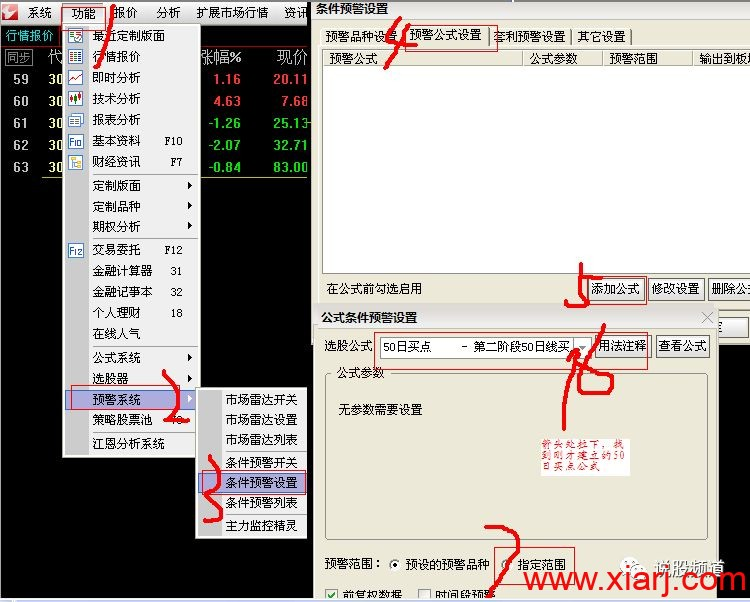8.选择范围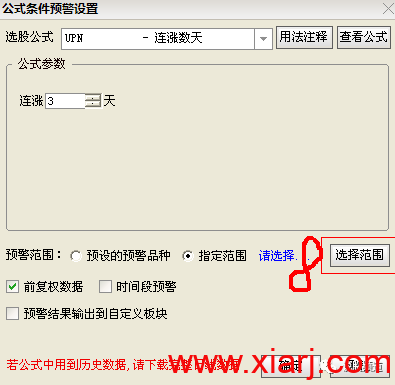9.选择刚才建立的自定义板块，每日第二阶段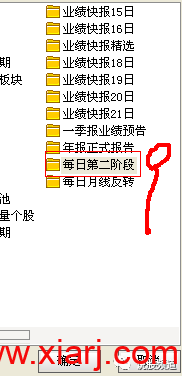10.建立完毕，如下图，点击确定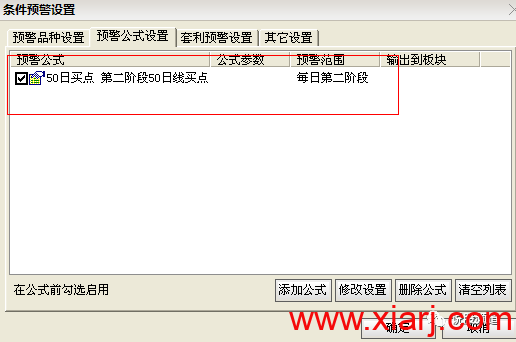11.选择是，就开启盘中预警功能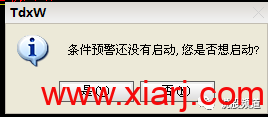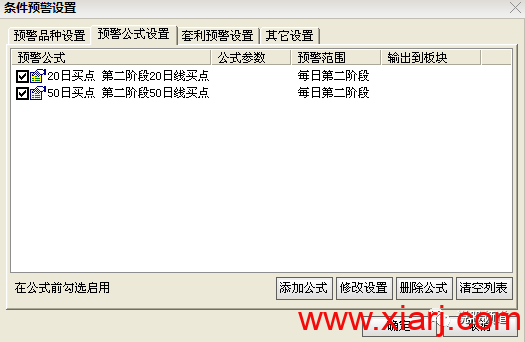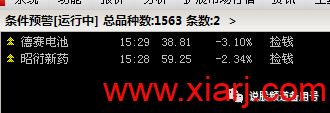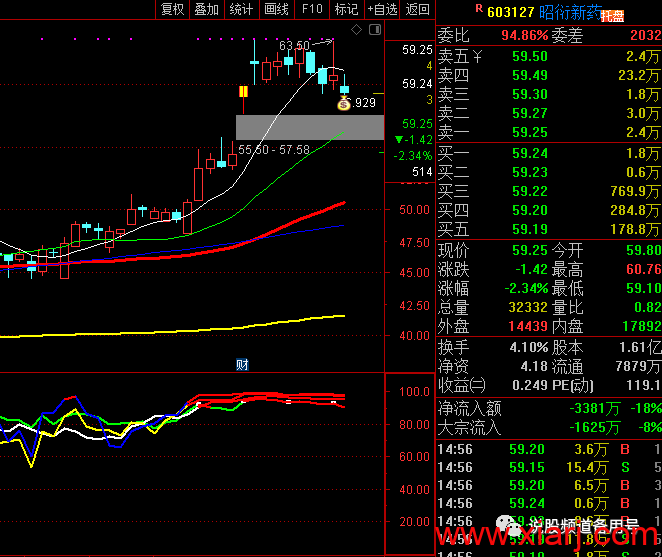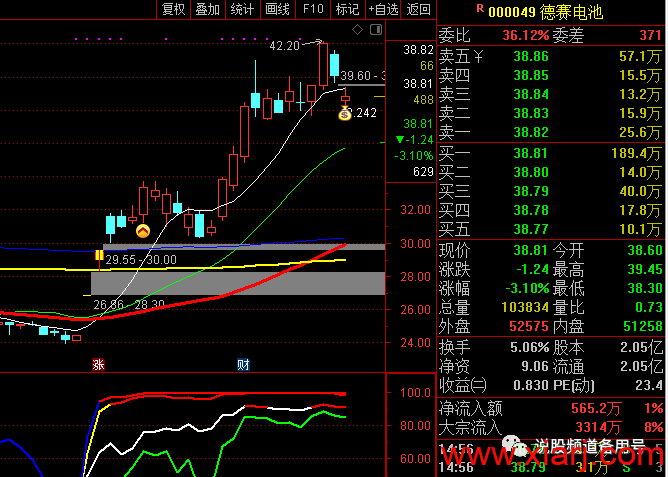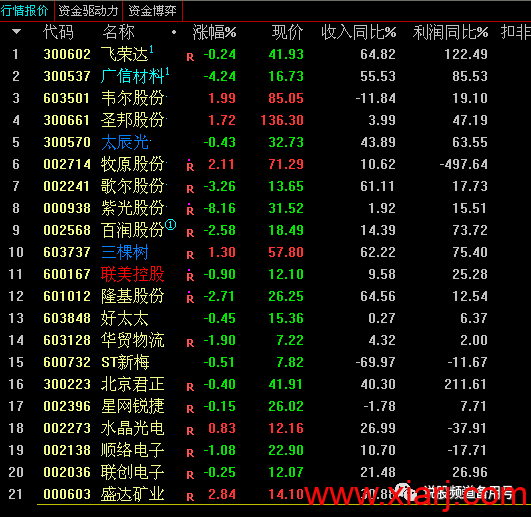### 评论列表(4)

1. 闽发论坛说道：

师傅领进门，修行在个人，博士已经过亿了